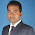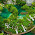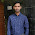## Measurements Instrumentation MCQ Questions Answers: Part-11

 The percentage limiting error, in the case of an instrument reading of 8.3V with a 0 to 150V voltmeter having a guaranteed accuracy of 1% full-scale reading is
A) 1.810%
B) 0.181%
C) 0.0018%
D) 18.10%

 The induction of  a high Q inductor can be measured by a
A) Schering Bridge
B) Hay Bridge
C) Maxwell Bridge
D) Wien Bridge

 If a DC voltmeter is made from an ammeter having a fed of 100μA then its sensitivity (in KΩ/V) will be
A) 1
B) 100
C) 10
D) 1000

Guidelines: Assertion (A) and Reason (R) type Questions 4 and 5:

(A) Both a and R are correct and R is explanation for A.
(B) Both a and R are correct and R is not explanation for A.
(C) A is true but R is false.
(D) A is false but R is true.

 A: The screen of a CRT is coated with phosphor on the inside.
R: Phosphor absorbs the KE of the bombarding electrons and re-emits energy at a frequency in the visible region.

  A: The Weign Bridge can be used for frequency measurements.
B: The Weign Bridge uses only capacitors and resistors.

 It is desired to convert a 0-1000A meter movement, with an internal resistance of 100 ohms, into a 0-100mA meter. The required value of shunt resistance is about
A) 0.1 ohms
B) 1 ohms
C)  99 ohms
D) 100 ohms

 Match List I with List II:
 List I List II (a) Megger (p) Measurement of loss angle in a dielectric (b) Spectrum analyzer (q) Measurement of frequency (c) Schering bridge (r) Measurement of insulation resistance (d) Digital counter (s) Measurement of harmonics

 In moving coil meters, damping is provided by

A) damping vane in the air tight chamber.
B) the aluminium frame of the coil.
C) eddy current disk.
D) The coil spring attached to the moving mechanism.

 A 0 to 300V voltmeter has a guaranteed accuracy of 1% of full scale reading. The voltage measured by the instrument is 83V. The percentage limiting error is
A) 95%
B) 4.85%
C) 3.62%
D)1.81%

 An Aryton shunt is used to make a D'Arsonval galvanometer into a
A) single range voltmeter
B) single range ammeter
C) multi range ammeter
D) multi range voltmeter
1:18 AM

#### 8 comments:

1.False answer in
 Match List I with List II:
List I List II
(a) Megger (p) Measurement of loss angle in a dielectric
(b) Spectrum analyzer (q) Measurement of frequency
(c) Schering bridge (r) Measurement of insulation resistance
(d) Digital counter (s) Measurement of harmonics

1.check out 9th answer...it is 3.6

2.check out the 9th one it is 3.6

2.Can anyone give me the explanation of question no.6 with calculations

3.Question no 6 dnt have answer

4.1.5.Please anyone explain questions. 9